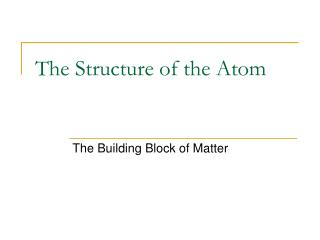DownloadDownload PresentationThe Structure of the Atom

# The Structure of the Atom

Download Presentation## The Structure of the Atom

- - - - - - - - - - - - - - - - - - - - - - - - - - - E N D - - - - - - - - - - - - - - - - - - - - - - - - - - -
##### Presentation Transcript

1. The Structure of the Atom The Building Block of Matter

2. Atoms are the smallest particles of an element that retains the chemical properties of that element • Parts: • Nucleus – small region in the center of the atom. (+ charged protons, = charged neutrons) • Electron Cloud – large region, compared to nucleus, where electrons are found.

3. Size of nucleus vs. electron cloud If an atom was the size of a sports stadium, the nucleus would be the size of a marble! Subatomic Particles: • Protons : + charge, relative mass = 1.007 atomic mass units (amu); round to 1 • Neutrons: = charge, relative mass = 1.009 atomic mass units (amu); round to 1 • Electrons: - charge, relative mass = 0.0005 atomic mass units (amu); round to 0 (not factored in when figuring total mass of an atom)

4. Structure of Subatomic Particles • Subatomic particles composed of fast moving points of energy called quarks Quark Calculations(for protons and neutrons) Each proton is 2 up quarks and 1 down quark 2(2/3) – 1(1/3) = 4/3 – 1/3 = 3/3 or +1 Each neutron is 2 down quarks and 1 up quark 2(-1/3) + 2/3 = 0 Each electron is composed of 2 down quarks 3(-1/3) = -1

5. Atomic Number • Number of protons in the nucleus (also the number of electrons) • Typically the number on top of the symbol on the Periodic Table. 13 Atomic Number 26.981

6. Mass Number • Number of protons + number of neutrons in an atom. • When solving for number of neutrons: round mass number properly, subtract atomic number from mass number.

7. Element Symbols • Hyphen Notation: Element symbol – Mass # (rounded) Example: Helium  He-4 • Nuclear Symbol: Superscript Mass Number, Subscript Atomic Number, Symbol Example: 4 He 2

8. IONS • IONS are atoms or groups of atoms with a positive or negative charge. • Taking away an electron from an atom gives a CATION with a positive charge • Adding an electron to an atom gives an ANION with a negative charge. • To tell the difference between an atom and an ion, look to see if there is a charge in the superscript! Examples: Na+ Ca+2 I- O-2 Na Ca I O

9. Forming Cations & Anions A CATION forms when an atom loses one or more electrons. An ANION forms when an atom gains one or more electrons Mg --> Mg2+ + 2 e- F + e- --> F-

10. Isotopes • Atoms with the same number of protons & electrons but a different number of neutrons. • They are the same element, but have different masses. (different mass number) • All isotopes are used to calculate atomic mass (reason why weight is a decimal). • Most elements consist of a mixture of isotopes.

11. 11B 10B Average Atomic Mass • Because of the existence of isotopes, the mass of a collection of atoms has an average value. • Boron is 20% 10B and 80% 11B. That is, 11B is 80 percent abundant on earth. • For boron average atomic mass = 0.20 (10 amu) + 0.80 (11 amu) = 10.8 amu

12. How to determine Average Atomic Mass • Determine Relative Abundance – % abundance divided by 100 • Determine weight of each isotope – multiply relative abundance by mass (keep all digits calculator gives you, no rounding yet) • Determine Average Atomic Mass - add up all individual weights, round to “2” decimal places

13. How to determine Percent Abundance • Use the following equation: Ave. Atomic Mass = (X)(Mass of 1st isotope)+(1-X)(Mass of 2nd isotope) • Solve for “X” • Answer will be a decimal • To solve for other isotope, do 1 – answer • Multiply both answers by 100 • Two answer should equal 100